# 十七、基于 DNN 模块的人脸检测与识别

• 基于不同方法的人脸检测
• 人脸预处理
• 从收集到的人脸训练机器学习算法
• 人脸识别
• 最后的润色

# 人脸检测与人脸识别简介

1. 人脸检测：这是在图像中定位人脸区域(以下截图中心附近的大矩形)的基本过程。 这一步并不关心这个人是谁，只关心它是一张人脸。
2. 人脸预处理：这是调整人脸图像，使其看起来更清晰、更接近其他人脸(以下截图中央上方的小灰度人脸)的最新流程。
3. 收集和学习人脸：这是一个保存许多预处理过的人脸(对于每个应该识别的人)，然后学习如何识别它们的过程。
4. 人脸识别：这是检查采集到的哪些人与摄像头中的人脸最相似的最新流程(下面截图右上角的一个小矩形)。

Note that the phrase face recognition is often used by the general public to refer to finding the positions of faces (that is, face detection, as described in step 1), but this book will use the formal definition of face recognition referring to step 4, and face detection referring to Step 1.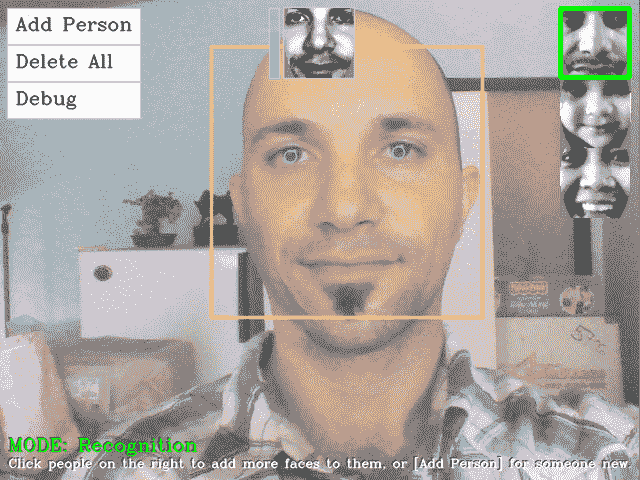# 人脸检测

OpenCV 实现了从 V3.4 到 V4.0 的深度学习，在这一章中，我们将展示如何使用单镜头多盒检测器和(SSD)算法进行人脸检测。

# 利用 OpenCV 级联分类器实现人脸检测

| 级联分类器的类型 | XML 文件名 | | 人脸检测器(默认) | haarcascade_frontalface_default.xml | | 人脸检测器(FAST HAAR) | haarcascade_frontalface_alt2.xml | | 人脸检测器(快速 LBP) | lbpcascade_frontalface.xml | | 侧面(侧视)人脸检测器 | haarcascade_profileface.xml | | 眼睛探测器(左右分开) | haarcascade_lefteye_2splits.xml | | 口腔探测仪 | haarcascade_mcs_mouth.xml | | 鼻部探测器 | haarcascade_mcs_nose.xml | | 全人探测器 | haarcascade_fullbody.xml |

# 加载用于目标或人脸检测的 Haar 或 LBP 检测器

    CascadeClassifier faceDetector;


    CascadeClassifier faceDetector;
try {
} catch (cv::Exception e) {}
if ( faceDetector.empty() ) {
cerr << "ERROR: Couldn't load Face Detector (";
cerr << faceCascadeFilename << ")!" << endl;
exit(1);
}


# 使用 Haar 或 LBP 分类器检测对象

1. 灰度颜色转换：人脸检测仅适用于灰度图像。 所以我们应该把彩色相机的画面转换成灰度。
2. 缩小相机图像：人脸检测的速度取决于输入图像的大小(对于大图像非常慢，但对于小图像很快)，但是即使在低分辨率下，检测仍然相当可靠。 因此，我们应该将摄像机图像缩小到更合理的大小(或者在检测器中使用较大的值minFeatureSize，如以下各节所述)。
3. 直方图均衡：人脸检测在弱光条件下不太可靠。 因此，需要对图像进行直方图均衡化，以提高图像的对比度和亮度。

    Mat gray;
if (img.channels() == 3) {
cvtColor(img, gray, COLOR_BGR2GRAY);
}
else if (img.channels() == 4) {
cvtColor(img, gray, COLOR_BGRA2GRAY);
}
else {
// Access the grayscale input image directly.
gray = img;
}


    const int DETECTION_WIDTH = 320;
// Possibly shrink the image, to run much faster.
Mat smallImg;
float scale = img.cols / (float) DETECTION_WIDTH;
if (img.cols > DETECTION_WIDTH) {
// Shrink the image while keeping the same aspect ratio.
int scaledHeight = cvRound(img.rows / scale);
resize(img, smallImg, Size(DETECTION_WIDTH, scaledHeight));
}
else {
// Access the input directly since it is already small.
smallImg = img;
}


    // Standardize the brightness & contrast, such as
// to improve dark images.
Mat equalizedImg;
equalizeHist(inputImg, equalizedImg);


# 检测人脸

• minFeatureSize：此参数确定我们关心的最小人脸大小，通常为 20x20 或 30x30 像素，但这取决于您的使用案例和图像大小。 如果您在网络摄像头或智能手机上执行人脸检测，其中人脸始终离摄像头很近，则可以将其放大到 80 x 80 以获得更快的检测速度，或者如果您想要检测较远的人脸，如与朋友一起在海滩上，则将其保留为 20 x 20。
• searchScaleFactor：此参数确定要查找多少个不同大小的人脸；通常情况下，如果检测良好，则为1.1；如果检测更快，则为1.2，这样就不会经常找到人脸。
• minNeighbors：此参数确定检测器应在多大程度上确定它已检测到人脸；它的值通常为 13，但如果您想要更可靠的人脸，即使没有检测到许多人脸，也可以将其设置得更高。
• flags：此参数允许您指定是查找所有面(默认)，还是仅查找最大的面(CASCADE_FIND_BIGGEST_OBJECT)。 如果你只寻找最大的脸，它应该跑得更快。 您还可以添加其他几个参数，使检测速度提高约 1%或 2%，如CASCADE_DO_ROUGH_SEARCHCASCADE_SCALE_IMAGE

    int flags = CASCADE_SCALE_IMAGE; // Search for many faces.
Size minFeatureSize(20, 20);     // Smallest face size.
float searchScaleFactor = 1.1f;  // How many sizes to search.
int minNeighbors = 4;            // Reliability vs many faces.

// Detect objects in the small grayscale image.
std::vector<Rect> faces;
faceDetector.detectMultiScale(img, faces, searchScaleFactor,
minNeighbors, flags, minFeatureSize);


    // Enlarge the results if the image was temporarily shrunk.
if (img.cols > scaledWidth) {
for (auto& object:objects ) {
object.x = cvRound(object.x * scale);
object.y = cvRound(object.y * scale);
object.width = cvRound(object.width * scale);
object.height = cvRound(object.height * scale);
}
}
// If the object is on a border, keep it in the image.
for (auto& object:objects) {
if (object.x < 0)
object.x = 0;
if (object.y < 0)
object.y = 0;
if (object.x + object.width > img.cols)
object.x = img.cols - object.width;
if (object.y + object.height > img.rows)
object.y = img.rows - object.height;
}


    int flags = CASCADE_FIND_BIGGEST_OBJECT |


WebcamFaceRec项目包括 OpenCV 的 Haar 或 LBP 探测器的包装，以便更容易在图像中找到人脸或眼睛，例如：

Rect faceRect;    // Stores the result of the detection, or -1\.
int scaledWidth = 320;     // Shrink the image before detection.
detectLargestObject(cameraImg, faceDetector, faceRect, scaledWidth);
if (faceRect.width > 0)
cout << "We detected a face!" << endl;


    // Access just the face within the camera image.
Mat faceImg = cameraImg(faceRect);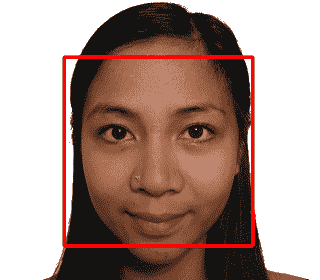# 利用 OpenCV 深度学习模块实现人脸检测

• Proto 文件或配置模型；在我们的示例中，该文件保存在data/deploy.prototxt
• 二进制训练模型，其中包含每个变量的权重；在我们的示例中，文件保存在data/res10_300x300_ssd_iter_140000_fp16.caffemodel

dnn::Net net = readNetFromCaffe("data/deploy.prototxt", "data/res10_300x300_ssd_iter_14000_fp16.caffemodel");


Mat inputBlob = blobFromImage(frame, 1.0, Size(300, 300), meanVal, false, false);


net.setInput(inputBlob);


Mat detection = net.forward();


# 人脸预处理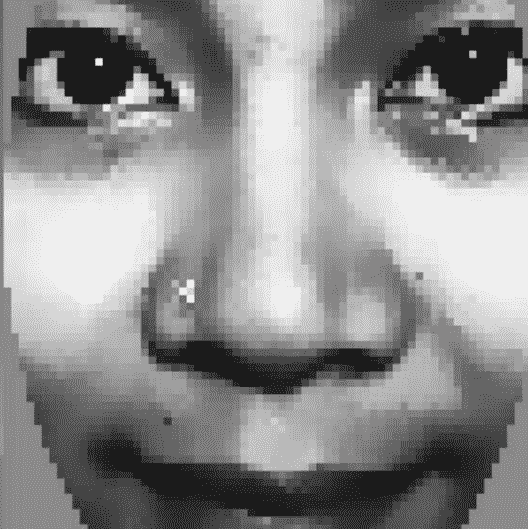# 眼睛检测

OpenCV v2.4 附带的一些预先训练的眼睛检测器可以检测眼睛是睁开的还是闭着的，而有些只能检测睁开的眼睛。

• haarcascade_mcs_lefteye.xml(andhaarcascade_mcs_righteye.xml)
• haarcascade_lefteye_2splits.xml(andhaarcascade_righteye_2splits.xml)

• haarcascade_eye.xml
• haarcascade_eye_tree_eyeglasses.xml

As the open or closed eye detectors specify which eye they are trained on, you need to use a different detector for the left and the right eye, whereas the detectors for just open eyes can use the same detector for left or right eyes. The haarcascade_eye_tree_eyeglasses.xml detector can detect the eyes if the person is wearing glasses, but is not reliable if they don't wear glasses. If the XML filename says left eye, it means the actual left eye of the person, so in the camera image it would normally appear on the right-hand side of the face, not on the left-hand side! The list of four eye detectors mentioned is ranked in approximate order from most reliable to least reliable, so if you know you don't need to find people with glasses, then the first detector is probably the best choice.

# 眼睛搜索区域

| 级联分类器 | EYE_SX | EYE_SY | EYE_SW | EYE_SH | | haarcascade_eye.xml | 0.16 | 0.26 | 0.30 | 0.28 | | haarcascade_mcs_lefteye.xml | 0.10 | 0.19 | 0.40 | 0.36 | | haarcascade_lefteye_2splits.xml | 0.12 | 0.17 | 0.37 | 0.36 |

    int leftX = cvRound(face.cols * EYE_SX);
int topY = cvRound(face.rows * EYE_SY);
int widthX = cvRound(face.cols * EYE_SW);
int heightY = cvRound(face.rows * EYE_SH);
int rightX = cvRound(face.cols * (1.0-EYE_SX-EYE_SW));

Mat topLeftOfFace = faceImg(Rect(leftX, topY, widthX, heightY));
Mat topRightOfFace = faceImg(Rect(rightX, topY, widthX, heightY));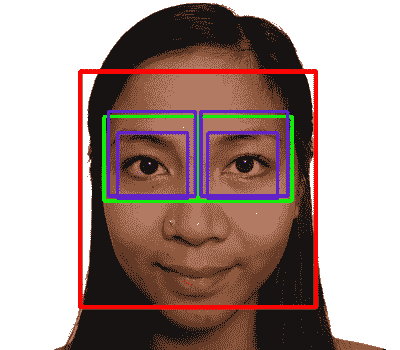| 级联分类器 | 可靠性 | 速度 | *发现眼睛 | 眼镜 | | haarcascade_mcs_lefteye.xml | 80% | 18 毫秒 | 打开或关闭 | 完全不 / 决不 / 不 | | haarcascade_lefteye_2splits.xml | 60% | 7 毫秒 | 打开或关闭 | 完全不 / 决不 / 不 | | haarcascade_eye.xml | 40% | 5 毫秒 | 仅打开 | 完全不 / 决不 / 不 | | haarcascade_eye_tree_eyeglasses.xml | 15% | 10 毫秒 | 仅打开 | 肯定的回答 / 赞成 / 是 |

Based on the table, it seems that when choosing an eye detector to use, you should decide whether you want to detect closed eyes or only open eyes. And remember that you can even use one eye detector, and if it does not detect an eye, then you can try with another one. For many tasks, it is useful to detect eyes whether they are open or closed, so if speed is not crucial, it is best to search with the mcs_*eye detector first, and if it fails, then search with the eye_2splits detector. But for face recognition, a person will appear quite different if their eyes are closed, so it is best to search with the plain haarcascade_eye detector first, and if it fails, then search with the haarcascade_eye_tree_eyeglasses detector.

    CascadeClassifier eyeDetector1("haarcascade_eye.xml");
...
Rect leftEyeRect;    // Stores the detected eye.
// Search the left region using the 1st eye detector.
detectLargestObject(topLeftOfFace, eyeDetector1, leftEyeRect,
topLeftOfFace.cols);
// If it failed, search the left region using the 2nd eye
// detector.
if (leftEyeRect.width <= 0)
detectLargestObject(topLeftOfFace, eyeDetector2,
leftEyeRect, topLeftOfFace.cols);
// Get the left eye center if one of the eye detectors worked.
Point leftEye = Point(-1,-1);
if (leftEyeRect.width <= 0) {
leftEye.x = leftEyeRect.x + leftEyeRect.width/2 + leftX;
leftEye.y = leftEyeRect.y + leftEyeRect.height/2 + topY;
}

// Do the same for the right eye
...

// Check if both eyes were detected.
if (leftEye.x >= 0 && rightEye.x >= 0) {
...
}


1. 几何变换和裁剪：此过程包括缩放、旋转和平移图像以使眼睛对齐，然后从人脸图像中移除前额、下巴、耳朵和背景。
2. 左右两侧的单独直方图均衡：此过程独立地标准化脸部左右两侧的亮度和对比度。
3. 平滑：此过程使用双边滤波器降低图像噪声。
4. 椭圆蒙版：椭圆蒙版从脸部图像中去除一些剩余的毛发和背景。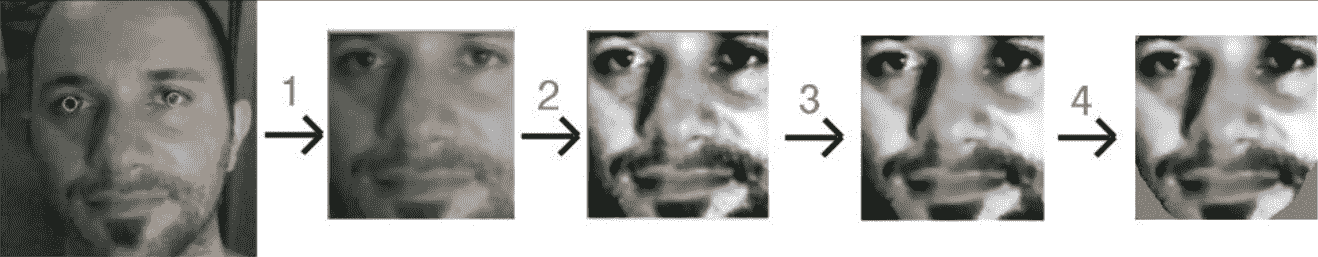# 几何变换

• 旋转面，使两只眼睛水平
• 缩放面，使两只眼睛之间的距离始终相同
• 平移面，使眼睛始终水平居中，并位于所需高度
• 裁剪人脸的外部，因为我们要裁剪图像背景、头发、额头、耳朵和下巴

    // Get the center between the 2 eyes.
Point2f eyesCenter;
eyesCenter.x = (leftEye.x + rightEye.x) * 0.5f;
eyesCenter.y = (leftEye.y + rightEye.y) * 0.5f;

// Get the angle between the 2 eyes.
double dy = (rightEye.y - leftEye.y);
double dx = (rightEye.x - leftEye.x);
double len = sqrt(dx*dx + dy*dy);

double angle = atan2(dy, dx) * 180.0/CV_PI;

// Hand measurements shown that the left eye center should
// ideally be roughly at (0.16, 0.14) of a scaled face image.
const double DESIRED_LEFT_EYE_X = 0.16;
const double DESIRED_RIGHT_EYE_X = (1.0f - 0.16);

// Get the amount we need to scale the image to be the desired
// fixed size we want.
const int DESIRED_FACE_WIDTH = 70;
const int DESIRED_FACE_HEIGHT = 70;
double desiredLen = (DESIRED_RIGHT_EYE_X - 0.16);
double scale = desiredLen * DESIRED_FACE_WIDTH / len;


    // Get the transformation matrix for the desired angle & size.
Mat rot_mat = getRotationMatrix2D(eyesCenter, angle, scale);
// Shift the center of the eyes to be the desired center.
double ex = DESIRED_FACE_WIDTH * 0.5f - eyesCenter.x;
double ey = DESIRED_FACE_HEIGHT * DESIRED_LEFT_EYE_Y -
eyesCenter.y;
rot_mat.at<double>(0, 2) += ex;
rot_mat.at<double>(1, 2) += ey;
// Transform the face image to the desired angle & size &
// position! Also clear the transformed image background to a
// default grey.
Mat warped = Mat(DESIRED_FACE_HEIGHT, DESIRED_FACE_WIDTH,
CV_8U, Scalar(128));
warpAffine(gray, warped, rot_mat, warped.size());


# 左右两侧分开的直方图均衡化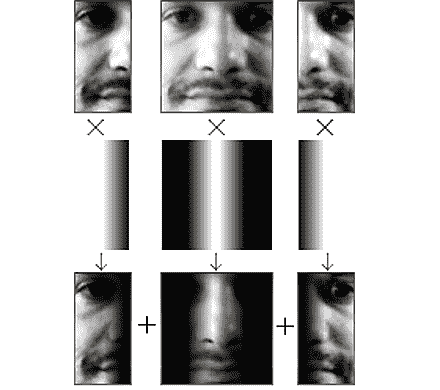int w = faceImg.cols;
int h = faceImg.rows;
Mat wholeFace;
equalizeHist(faceImg, wholeFace);
int midX = w/2;
Mat leftSide = faceImg(Rect(0,0, midX,h));
Mat rightSide = faceImg(Rect(midX,0, w-midX,h));
equalizeHist(leftSide, leftSide);
equalizeHist(rightSide, rightSide);


    for (int y=0; y<h; y++) {
for (int x=0; x<w; x++) {
int v;
if (x < w/4) {
// Left 25%: just use the left face.
v = leftSide.at<uchar>(y,x);
}
else if (x < w*2/4) {
// Mid-left 25%: blend the left face & whole face.
int lv = leftSide.at<uchar>(y,x);
int wv = wholeFace.at<uchar>(y,x);
// Blend more of the whole face as it moves
// further right along the face.
float f = (x - w*1/4) / (float)(w/4);
v = cvRound((1.0f - f) * lv + (f) * wv);
}
else if (x < w*3/4) {
// Mid-right 25%: blend right face & whole face.
int rv = rightSide.at<uchar>(y,x-midX);
int wv = wholeFace.at<uchar>(y,x);
// Blend more of the right-side face as it moves
// further right along the face.
float f = (x - w*2/4) / (float)(w/4);
v = cvRound((1.0f - f) * wv + (f) * rv);
}
else {
// Right 25%: just use the right face.
v = rightSide.at<uchar>(y,x-midX);
}
faceImg.at<uchar>(y,x) = v;
} // end x loop
} //end y loop


# 光滑的 / 平滑的 / 醇和的 / 不苦的

    Mat filtered = Mat(warped.size(), CV_8U);
bilateralFilter(warped, filtered, 0, 20.0, 2.0);


# 椭圆掩模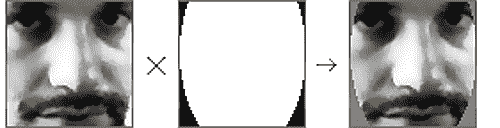// Draw a black-filled ellipse in the middle of the image.
// First we initialize the mask image to white (255).
Mat mask = Mat(warped.size(), CV_8UC1, Scalar(255));
double dw = DESIRED_FACE_WIDTH;
double dh = DESIRED_FACE_HEIGHT;
Point faceCenter = Point( cvRound(dw * 0.5),
cvRound(dh * 0.4) );
Size size = Size( cvRound(dw * 0.5), cvRound(dh * 0.8) );
ellipse(mask, faceCenter, size, 0, 0, 360, Scalar(0),
CV_FILLED);

// Apply the elliptical mask on the face, to remove corners.
// Sets corners to gray, without touching the inner face.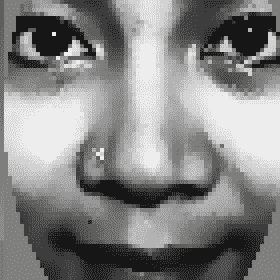# 收集人脸并从中学习

One way to obtain a good training set that will cover many different real-world conditions is for each person to rotate their head from looking left, to up, to right, to down, then looking directly straight. Then, the person tilts their head sideways and then up and down, while also changing their facial expression, such as alternating between smiling, looking angry, and having a neutral face. If each person follows a routine such as this while collecting faces, then there is a much better chance of recognizing everyone in real-world conditions. For even better results, it should be performed again with one or two more locations or directions, such as by turning the camera around 180 degrees, walking in the opposite direction, and then repeating the whole routine, so that the training set would include many different lighting conditions.

# 采集预处理后的人脸进行训练

    // Check how long since the previous face was added.
double current_time = (double)getTickCount();
double timeDiff_seconds = (current_time -
old_time) / getTickFrequency();


    double getSimilarity(const Mat A, const Mat B) {
// Calculate the L2 relative error between the 2 images.
double errorL2 = norm(A, B, CV_L2);
// Scale the value since L2 is summed across all pixels.
double similarity = errorL2 / (double)(A.rows * A.cols);
return similarity;
}

...

// Check if this face looks different from the previous face.
double imageDiff = MAX_DBL;
if (old_prepreprocessedFaceprepreprocessedFace.data) {
imageDiff = getSimilarity(preprocessedFace,
old_prepreprocessedFace);
}


    // Only process the face if it's noticeably different from the
// previous frame and there has been a noticeable time gap.
if ((imageDiff > 0.3) && (timeDiff_seconds > 1.0)) {
// Also add the mirror image to the training set.
Mat mirroredFace;
flip(preprocessedFace, mirroredFace, 1);

// Add the face & mirrored face to the detected face lists.
preprocessedFaces.push_back(preprocessedFace);
preprocessedFaces.push_back(mirroredFace);
faceLabels.push_back(m_selectedPerson);
faceLabels.push_back(m_selectedPerson);

// Keep a copy of the processed face,
// to compare on next iteration.
old_prepreprocessedFace = preprocessedFace;
old_time = current_time;
}


    // Get access to the face region-of-interest.
Mat displayedFaceRegion = displayedFrame(faceRect);
// Add some brightness to each pixel of the face region.
displayedFaceRegion += CV_RGB(90,90,90);


# 从采集到的人脸训练人脸识别系统

• 特征脸(也称为主成分分析)(PCA)
• FisherFaces(也称为线性判别分析)(LDA)
• 其他经典的人脸识别算法(许多都可以在http://www.facerec.org/algorithms/上找到)
• 较新的人脸识别算法在最近的计算机视觉研究论文中(如http://www.cvpapers.com/的 CCVPR 和 ICCV)，因为每年发表的人脸识别论文数以百计

    vector<string> algorithms;
Algorithm::getList(algorithms);
cout << "Algorithms: " << algorithms.size() << endl;
for (auto& algorithm:algorithms) {
cout << algorithm << endl;
}


• FaceRecognizer.Eigenfaces：特征脸，也被称为 PCA，1991 年首次被土耳其人和宾特兰人使用
• FaceRecognizer.Fisherfaces渔脸，又称 LDA，由 Belhumeur、Hespanha 和 Kriegman 于 1997 年发明
• FaceRecognizer.LBPH：局部二进制模式直方图，由 Ahonen、Hahad 和 Pietikäinen 于 2004 年发明

More information on these face recognition algorithm implementations can be found with documentation, samples, and Python equivalents for each of them on Philipp Wagner's websites (http://bytefish.de/blog and http://bytefish.de/dev/libfacerec/).

    // Load the "contrib" module is dynamically at runtime.
bool haveContribModule = initModule_contrib();
if (!haveContribModule) {
cerr << "ERROR: The 'contrib' module is needed for ";
cerr << "FaceRecognizer but hasn't been loaded to OpenCV!";
cerr << endl;
exit(1);
}


    string facerecAlgorithm = "FaceRecognizer.Fisherfaces";
Ptr<FaceRecognizer> model;
// Use OpenCV's new FaceRecognizer in the "contrib" module:
model = Algorithm::create<FaceRecognizer>(facerecAlgorithm);
if (model.empty()) {
cerr << "ERROR: The FaceRecognizer [" << facerecAlgorithm;
cerr << "] is not available in your version of OpenCV. ";
exit(1);
}


    // Do the actual training from the collected faces.
model->train(preprocessedFaces, faceLabels);


# 查看学到的知识

    // Convert the matrix row or column (float matrix) to a
// rectangular 8-bit image that can be displayed or saved.
// Scales the values to be between 0 to 255\.
Mat getImageFrom1DFloatMat(const Mat matrixRow, int height)
{
// Make a rectangular shaped image instead of a single row.
Mat rectangularMat = matrixRow.reshape(1, height);
// Scale the values to be between 0 to 255 and store them
// as a regular 8-bit uchar image.
Mat dst;
normalize(rectangularMat, dst, 0, 255, NORM_MINMAX,
CV_8UC1);
return dst;
}


    Mat img = ...;
printMatInfo(img, "My Image");


My Image: 640w480h 3ch 8bpp, range[79,253][20,58][18,87]


It is also possible to print the actual contents of an image or matrix by using the printMat() function instead of the printMatInfo() function. This is quite handy for viewing matrices and multichannel-float matrices, as these can be quite tricky to view for beginners. The ImageUtils code is mostly for OpenCV's C interface, but is gradually including more of the C++ interface over time. The most recent version can be found at http://shervinemami.info/openCV.html.

# 平均脸部

    Mat averageFace = model->get<Mat>("mean");
printMatInfo(averageFace, "averageFace (row)");
// Convert a 1D float row matrix to a regular 8-bit image.
averageFace = getImageFrom1DFloatMat(averageFace, faceHeight);
printMatInfo(averageFace, "averageFace");
imshow("averageFace", averageFace);


 averageFace (row): 4900w1h 1ch 64bpp, range[5.21,251.47]
averageFace: 70w70h 1ch 8bpp, range[0,255]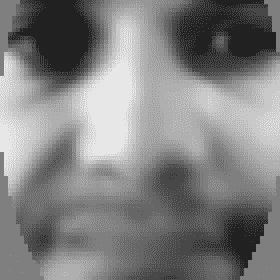# 特征值、特征面和鱼子面

    Mat eigenvalues = model->get<Mat>("eigenvalues");
printMat(eigenvalues, "eigenvalues");


    eigenvalues: 1w18h 1ch 64bpp, range[4.52e+04,2.02836e+06]
2.03e+06
1.09e+06
5.23e+05
4.04e+05
2.66e+05
2.31e+05
1.85e+05
1.23e+05
9.18e+04
7.61e+04
6.91e+04
4.52e+04


    eigenvalues: 2w1h 1ch 64bpp, range[152.4,316.6]
317, 152


   // Get the eigenvectors
Mat eigenvectors = model->get<Mat>("eigenvectors");
printMatInfo(eigenvectors, "eigenvectors");

// Show the best 20 Eigenfaces
for (int i = 0; i < min(20, eigenvectors.cols); i++) {
// Create a continuous column vector from eigenvector #i.
Mat eigenvector = eigenvectors.col(i).clone();

Mat eigenface = getImageFrom1DFloatMat(eigenvector,
faceHeight);
imshow(format("Eigenface%d", i), eigenface);
}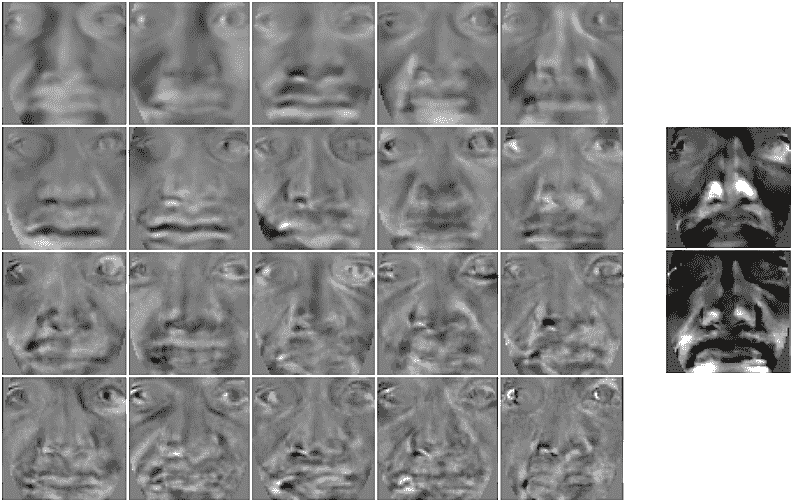# 人脸识别技术--从人脸识别人

    int identity = model->predict(preprocessedFace);


# 人脸验证-确认这就是被认领的人

OpenCV 的FaceRecognizer类可以在调用predict()函数时返回置信度度量，但不幸的是，置信度度量只是基于特征子空间中的距离，因此不是很可靠。 我们将使用的方法是使用第个特征向量和第二个特征值来重建人脸图像，并将重建的图像与输入图像进行比较。 如果该人的许多人脸包括在训练集中，则根据学习的特征向量和特征值进行重建应该工作得相当好，但是如果该人在训练集中没有任何人脸(或者没有任何具有与测试图像相似的光照和人脸表情的人脸)，则重建的人脸看起来将与输入人脸非常不同，这表明它可能是未知的人脸。

    // Get some required data from the FaceRecognizer model.
Mat eigenvectors = model->get<Mat>("eigenvectors");
Mat averageFaceRow = model->get<Mat>("mean");

// Project the input image onto the eigenspace.
Mat projection = subspaceProject(eigenvectors, averageFaceRow,
preprocessedFace.reshape(1,1));

// Generate the reconstructed face back from the eigenspace.
Mat reconstructionRow = subspaceReconstruct(eigenvectors,
averageFaceRow, projection);

// Make it a rectangular shaped image instead of a single row.
Mat reconstructionMat = reconstructionRow.reshape(1,
faceHeight);

// Convert the floating-point pixels to regular 8-bit uchar.
Mat reconstructedFace = Mat(reconstructionMat.size(), CV_8U);
reconstructionMat.convertTo(reconstructedFace, CV_8U, 1, 0);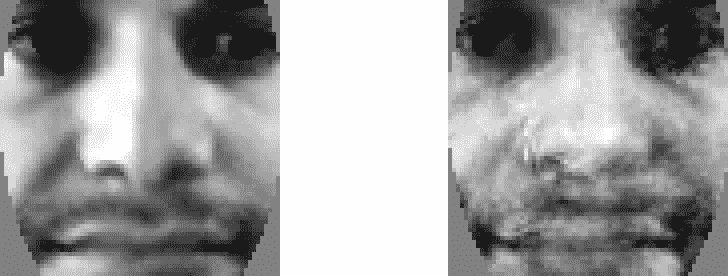similarity = getSimilarity(preprocessedFace, reconstructedFace);
if (similarity > UNKNOWN_PERSON_THRESHOLD) {
identity = -1;    // Unknown person.
}


# 点睛之笔-保存和加载文件

model->save("trainedModel.yml");


    string facerecAlgorithm = "FaceRecognizer.Fisherfaces";
model = Algorithm::create<FaceRecognizer>(facerecAlgorithm);
Mat labels;
try {
labels = model->get<Mat>("labels");
} catch (cv::Exception &e) {}
if (labels.rows <= 0) {
cerr << "ERROR: Couldn't load trained data from "
"[trainedModel.yml]!" << endl;
exit(1);
}


# 画龙点睛-打造漂亮的交互式图形用户界面

• 启动：此状态加载并初始化数据和网络摄像头。
• 检测：此状态检测人脸并对其进行预处理，直到用户单击添加人员按钮。
• Collection：此状态收集当前用户的面孔，直到用户单击窗口中的任何位置。 这也显示了每个人的最新面孔。 用户单击现有人员之一或单击添加人员按钮，即可为不同的人员收集人脸。
• 训练：在这种状态下，系统在所有收集的人的所有收集的人脸的帮助下进行训练。
• 识别：这包括突出显示被识别的人并显示置信度。 用户单击其中一个人员或单击添加人员按钮返回到模式 2(集合)。

# 绘制 GUI 元素

    // Draw text into an image. Defaults to top-left-justified
// text, so give negative x coords for right-justified text,
// and/or negative y coords for bottom-justified text.
// Returns the bounding rect around the drawn text.
Rect drawString(Mat img, string text, Point coord, Scalar
color, float fontScale = 0.6f, int thickness = 1,
int fontFace = FONT_HERSHEY_COMPLEX);


    string msg = "Click [Add Person] when ready to collect faces.";
// Draw it as black shadow & again as white text.
float txtSize = 0.4;
int BORDER = 10;
drawString (displayedFrame, msg, Point(BORDER, -BORDER-2),
CV_RGB(0,0,0), txtSize);
Rect rcHelp = drawString(displayedFrame, msg, Point(BORDER+1,
-BORDER-1), CV_RGB(255,255,255), txtSize);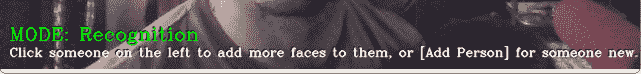// Draw a GUI button into the image, using drawString().
// Can give a minWidth to have several buttons of same width.
// Returns the bounding rect around the drawn button.
Rect drawButton(Mat img, string text, Point coord,
int minWidth = 0)
{
const int B = 10;
Point textCoord = Point(coord.x + B, coord.y + B);
// Get the bounding box around the text.
Rect rcText = drawString(img, text, textCoord,
CV_RGB(0,0,0));
// Draw a filled rectangle around the text.
Rect rcButton = Rect(rcText.x - B, rcText.y - B,
rcText.width + 2*B, rcText.height + 2*B);
// Set a minimum button width.
if (rcButton.width < minWidth)
rcButton.width = minWidth;
// Make a semi-transparent white rectangle.
Mat matButton = img(rcButton);
matButton += CV_RGB(90, 90, 90);
// Draw a non-transparent white border.
rectangle(img, rcButton, CV_RGB(200,200,200), 1, LINE_AA);

// Draw the actual text that will be displayed.
drawString(img, text, textCoord, CV_RGB(10,55,20));

return rcButton;
}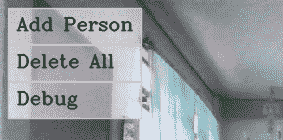# 启动模式

    // Create a GUI window for display on the screen.
namedWindow(windowName);

// Call "onMouse()" when the user clicks in the window.
setMouseCallback(windowName, onMouse, 0);

// Set the camera resolution. Only works for some systems.
videoCapture.set(CAP_PROP_FRAME_WIDTH, 640);
videoCapture.set(CAP_PROP_FRAME_HEIGHT, 480);

// We're already initialized, so let's start in Detection mode.
m_mode = MODE_DETECTION;


# 检测模式

• Mat preprocessedFace：预处理的人脸(如果检测到人脸和眼睛 )
• Rect faceRect：检测到的人脸区域坐标
• Point leftEyerightEye：检测到的左眼和右眼中心坐标

    bool gotFaceAndEyes = false;
if (preprocessedFace.data)
gotFaceAndEyes = true;

if (faceRect.width > 0) {
// Draw an anti-aliased rectangle around the detected face.
rectangle(displayedFrame, faceRect, CV_RGB(255, 255, 0), 2,
CV_AA);

// Draw light-blue anti-aliased circles for the 2 eyes.
Scalar eyeColor = CV_RGB(0,255,255);
if (leftEye.x >= 0) {   // Check if the eye was detected
circle(displayedFrame, Point(faceRect.x + leftEye.x,
faceRect.y + leftEye.y), 6, eyeColor, 1, LINE_AA);
}
if (rightEye.x >= 0) {   // Check if the eye was detected
circle(displayedFrame, Point(faceRect.x + rightEye.x,
faceRect.y + rightEye.y), 6, eyeColor, 1, LINE_AA);
}
}


    int cx = (displayedFrame.cols - faceWidth) / 2;
if (preprocessedFace.data) {
// Get a BGR version of the face, since the output is BGR.
Mat srcBGR = Mat(preprocessedFace.size(), CV_8UC3);
cvtColor(preprocessedFace, srcBGR, COLOR_GRAY2BGR);

// Get the destination ROI.
Rect dstRC = Rect(cx, BORDER, faceWidth, faceHeight);
Mat dstROI = displayedFrame(dstRC);

// Copy the pixels from src to dst.
srcBGR.copyTo(dstROI);
}
// Draw an anti-aliased border around the face.
rectangle(displayedFrame, Rect(cx-1, BORDER-1, faceWidth+2,
faceHeight+2), CV_RGB(200,200,200), 1, LINE_AA);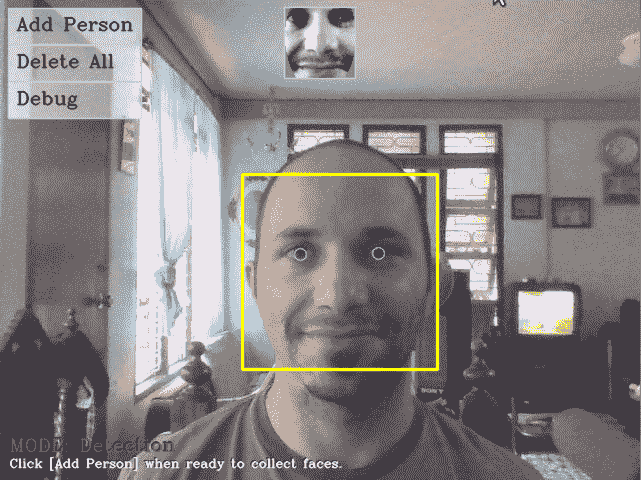# 采集模式

    // Keep a reference to the latest face of each person.
m_latestFaces[m_selectedPerson] = preprocessedFaces.size() - 2;


    m_gui_faces_left = displayedFrame.cols - BORDER - faceWidth;
m_gui_faces_top = BORDER;
for (int i=0; i<m_numPersons; i++) {
int index = m_latestFaces[i];
if (index >= 0 && index < (int)preprocessedFaces.size()) {
Mat srcGray = preprocessedFaces[index];
if (srcGray.data) {
// Get a BGR face, since the output is BGR.
Mat srcBGR = Mat(srcGray.size(), CV_8UC3);
cvtColor(srcGray, srcBGR, COLOR_GRAY2BGR);

// Get the destination ROI
int y = min(m_gui_faces_top + i * faceHeight,
displayedFrame.rows - faceHeight);
Rect dstRC = Rect(m_gui_faces_left, y, faceWidth,
faceHeight);
Mat dstROI = displayedFrame(dstRC);

// Copy the pixels from src to dst.
srcBGR.copyTo(dstROI);
}
}
}


    if (m_mode == MODE_COLLECT_FACES) {
if (m_selectedPerson >= 0 &&
m_selectedPerson < m_numPersons) {
int y = min(m_gui_faces_top + m_selectedPerson *
faceHeight, displayedFrame.rows - faceHeight);
Rect rc = Rect(m_gui_faces_left, y, faceWidth, faceHeight);
rectangle(displayedFrame, rc, CV_RGB(255,0,0), 3, LINE_AA);
}
}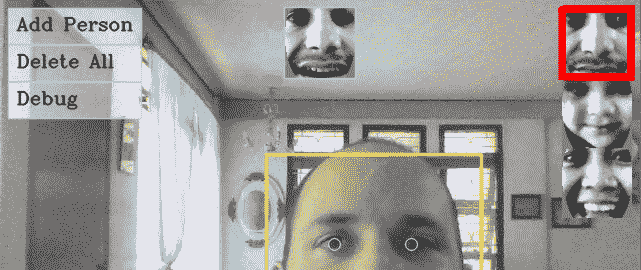# 培训模式

    // Check if there is enough data to train from.
bool haveEnoughData = true;
if (!strcmp(facerecAlgorithm, "FaceRecognizer.Fisherfaces")) {
if ((m_numPersons < 2) ||
(m_numPersons == 2 && m_latestFaces < 0) ) {
cout << "Fisherfaces needs >= 2 people!" << endl;
haveEnoughData = false;
}
}
if (m_numPersons < 1 || preprocessedFaces.size() <= 0 ||
preprocessedFaces.size() != faceLabels.size()) {
cout << "Need data before it can be learnt!" << endl;
haveEnoughData = false;
}

if (haveEnoughData) {
// Train collected faces using Eigenfaces or Fisherfaces.
model = learnCollectedFaces(preprocessedFaces, faceLabels,
facerecAlgorithm);

// Now that training is over, we can start recognizing!
m_mode = MODE_RECOGNITION;
}
else {
// Not enough training data, go back to Collection mode!
m_mode = MODE_COLLECT_FACES;
}


# 识别模式

    int cx = (displayedFrame.cols - faceWidth) / 2;
Point ptBottomRight = Point(cx - 5, BORDER + faceHeight);
Point ptTopLeft = Point(cx - 15, BORDER);

// Draw a gray line showing the threshold for "unknown" people.
Point ptThreshold = Point(ptTopLeft.x, ptBottomRight.y -
(1.0 - UNKNOWN_PERSON_THRESHOLD) * faceHeight);
rectangle(displayedFrame, ptThreshold, Point(ptBottomRight.x,
ptThreshold.y), CV_RGB(200,200,200), 1, CV_AA);

// Crop the confidence rating between 0 to 1 to fit in the bar.
double confidenceRatio = 1.0 - min(max(similarity, 0.0), 1.0);
Point ptConfidence = Point(ptTopLeft.x, ptBottomRight.y -
confidenceRatio * faceHeight);

// Show the light-blue confidence bar.
rectangle(displayedFrame, ptConfidence, ptBottomRight,
CV_RGB(0,255,255), CV_FILLED, CV_AA);

// Show the gray border of the bar.
rectangle(displayedFrame, ptTopLeft, ptBottomRight,
CV_RGB(200,200,200), 1, CV_AA);


    if (identity >= 0 && identity < 1000) {
int y = min(m_gui_faces_top + identity * faceHeight,
displayedFrame.rows - faceHeight);
Rect rc = Rect(m_gui_faces_left, y, faceWidth, faceHeight);
rectangle(displayedFrame, rc, CV_RGB(0,255,0), 3, CV_AA);
}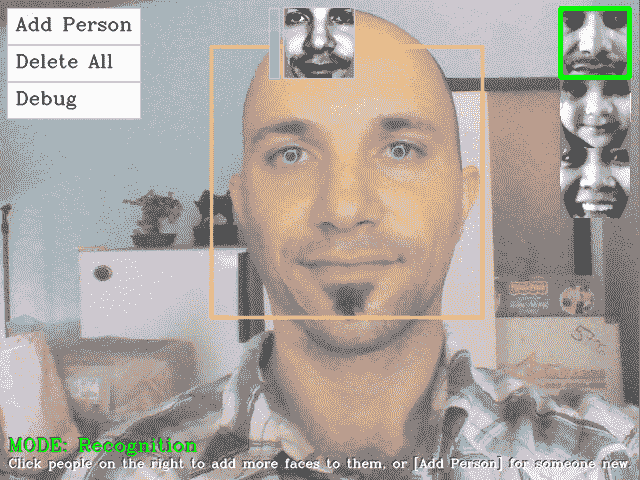# 检查和处理鼠标点击

    void onMouse(int event, int x, int y, int, void*)
{
if (event != CV_EVENT_LBUTTONDOWN)
return;

Point pt = Point(x,y);

... (handle mouse clicks)
...
}


    if (pt.inside(m_btnAddPerson)) {
// Ensure there isn't a person without collected faces.
if ((m_numPersons==0) ||
(m_latestFaces[m_numPersons-1] >= 0)) {
m_numPersons++ ;
m_latestFaces.push_back(-1);
}
m_selectedPerson = m_numPersons - 1;
m_mode = MODE_COLLECT_FACES;
}


    else if (pt.inside(m_btnDebug)) {
m_debug = !m_debug;
}


    else {
// Check if the user clicked on a face from the list.
int clickedPerson = -1;
for (int i=0; i<m_numPersons; i++) {
if (m_gui_faces_top >= 0) {
Rect rcFace = Rect(m_gui_faces_left,
m_gui_faces_top + i * faceHeight, faceWidth, faceHeight);
if (pt.inside(rcFace)) {
clickedPerson = i;
break;
}
}
}
// Change the selected person, if the user clicked a face.
if (clickedPerson >= 0) {
// Change the current person & collect more photos.
m_selectedPerson = clickedPerson;
m_mode = MODE_COLLECT_FACES;
}
// Otherwise they clicked in the center.
else {
// Change to training mode if it was collecting faces.
if (m_mode == MODE_COLLECT_FACES) {
m_mode = MODE_TRAINING;
}
}
}


# 参考文献

• 使用简单特征的增强级联的快速目标检测P.Viola 和 M.J.JonesIEEE 关于 CVPR 2001第 1 卷第 511-518 页的论文集，第 1 卷第 511-518 页第 1 卷第 511-518 页
• 一组扩展的用于快速目标检测的类 Haar 特征R.Lienhart 和 J.MaydtICIP 2002 年 IEEE 会议论文集第 1 卷第 900-903 页
• 使用局部二值模式的人脸描述：在人脸识别中的应用T.Ahonen，A.Hahad 和 M.PietikäinenIEEE Pami 2006 论文集第 28 卷第 12 期第 2037-2041 页
• 学习 OpenCV：使用 OpenCV 库的计算机视觉G.Bradski 和 A.Kaehler第 186-190 页O‘Reilly Media
• 用于识别的特征脸M.Turk 和 A.Pentland认知神经科学杂志 3第 71-86 页
• 特征脸与渔夫脸：使用类特定线性投影的识别P.N.Belhumeur，J.Hespanha 和 D.KriegmanIEEE 关于 Pami 1997 的会议论文集第 19 卷问题 7第 711-720 页Pami 1997第 19 卷问题 7第 711-720 页
• 基于局部二值模式的人脸识别T.Ahonen，A.Hahad 和 M.Pietikäinen计算机视觉-ECCV 2004第 469-48 页